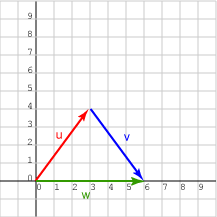If   g   =   (1, 1)T,   what is   | g |   ?

Plugging into the formula:

```| g |   =   √( 12 + 12 )

=   √2.0

=   1.414213562373
```

Notice that the length is NOT 1.0, nor 2.0. People sometimes make that mistake.

# Demonstration the Triangle InequalityRemember from the previous chapter that the length of the sum of two vectors is less than or equal to the sum of the lengths of the two vectors:

 length of ( u + v )  <=  length of ( u ) + length of ( v )

In our new notation:

 | u + v |  <=   | u | + | v |

Consider the vectors represented by:

```u   =   (3, 4)T
v   =   (3, -4)T
w   =   u + v = (6, 0)T
```

The picture shows how the triangle inequality works for this example. The sum of the red and the blue lengths is less than the green length. But does this work numerically?

### QUESTION 6:

What is | u |, | v |, and | w | ?